# Catch up on the latest AI articles# Diffusion Policy : Diffusion Models For Robots! When Robots Can Make Pizza!

3 main points
✔️ Formulate imitation learning with a diffusion model
✔️ Responds to multimodal and discrete cases and stabilizes learning compared to conventional methods
✔️ Improves success rate by 46.9% on average in simulations and real experiments

(Submitted on 7 Mar 2023 (v1), last revised 1 Jun 2023 (this version, v4))
Comments: Project website: this https URL
Subjects: Robotics (cs.RO)The images used in this article are from the paper, the introductory slides, or were created based on them.

## Introduction

There are many types of research in the field of robot learning, and one of the most actively studied methods is imitation learning. Imitation learning is a method to learn strategies from data obtained by an expert such as a human actually operating a robot. Due to its characteristics, imitation learning has advantages over other methods, such as no need for reward design, which is a problem in reinforcement learning, and no sim2real problem if demonstration data from an actual robot is used. In this article, we introduce the latest research on imitation learning, which has greatly improved performance compared to conventional methods.

The authors of the original paper have a website where you can see the robot in action and images of the method.

## Existing Research and Issues

Imitation learning-based methods have found it difficult to address the following two problems.

### Discontinuities and MultiModalities

The figure below shows a task in which the robot arm separates the yellow and blue blocks and places them in their respective goals.

Discreteness is a case in which the sequence of actions must be switched discretely, which in this case corresponds to the characteristic of a task in which the goal is switched every time. Multimodality is a case in which there are multiple ways to achieve a goal. In this task, the blocks to be moved can be either blue or yellow, and there are multiple possible processes to achieve the goal.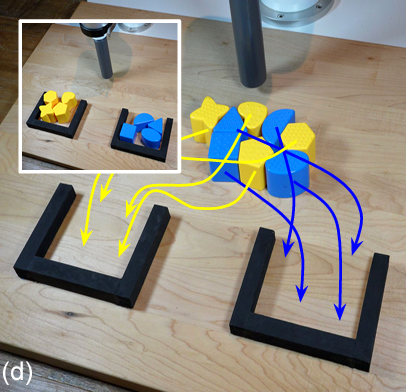### Implicit Policy and its Problems

Implicit Policy is a new way to deal with this problem. The conventional method is called Explicit Policy, which cannot deal with the above two problems because its policy is $\boldsymbol{a} = F_{\theta}(\boldsymbol{o})$ and the relationship between observation and action is expressed as a continuous function.

In contrast, Implicit Policy defines an energy-based model (EBM), $\boldsymbol{a}=\underset{\boldsymbol{a}}{\mathrm{argmin}} \hspace{2pt}E_{\theta}(\boldsymbol{o}, \boldsymbol{a})$. Implicit Policy is able to cope with discreteness and multimodality as shown in the figure below because it determines the action that minimizes the EBM, while Explicit Policy tries to approach the trajectory given by the human in a continuous model. Implicit Policy is able to cope with discreteness and multimodality, as shown in the figure below.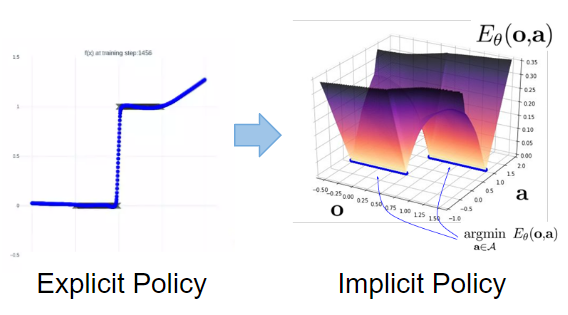However, Implicit Policy also has a problem: learning is not stable. The probability of determining an action is expressed as $p_{\theta}(\boldsymbol{a}|\boldsymbol{o}) = \dfrac{e^{-E_{\theta}(\boldsymbol{o}, \boldsymbol{a})}}{z(\boldsymbol{o}, \theta)}$, the loss function is expressed as $z(\boldsymbol{o}, \theta)$, which is a sampling approximation of $L_{infoNCE}=-\mathrm{log}\left(\dfrac{e^{-E_{\theta}(\boldsymbol{o}, \boldsymbol{a})}}{e^{-E_{\theta}(\boldsymbol{o}, \boldsymbol{a})} + \color{red}{\sum_{j=1}^{N_{neg}}} e^{-E_{\theta}(\boldsymbol{o}, \tilde{\boldsymbol{a}}^j)}}\right)$ is computed.

However, $z(\boldsymbol{o}, \theta)$ is a sampling approximation, which is a destabilizing factor for learning.

## Proposed Method

The Diffusion Policy was proposed to cope with these discreteness and multimodality and to stabilize learning.

### Diffusion Policy

Using the Difusion model, we formulate the followingBased on the Diffusion Process, $\boldsymbol{A}_t$ is the robot's behavior and $\boldsymbol{O}_t$ is the embedding of the observed information. The $\boldsymbol{A}_t^0$ is finally generated based on the Diffusion Process (see the left figure below). The system is a predictive closed-loop system, which generates an action sequence after obtaining an observation sequence at regular intervals. This contributes to smooth control sequences and robustness against disturbances (center of the figure below). The architecture of action generation is verified using both CNN and Transformer (right figure below).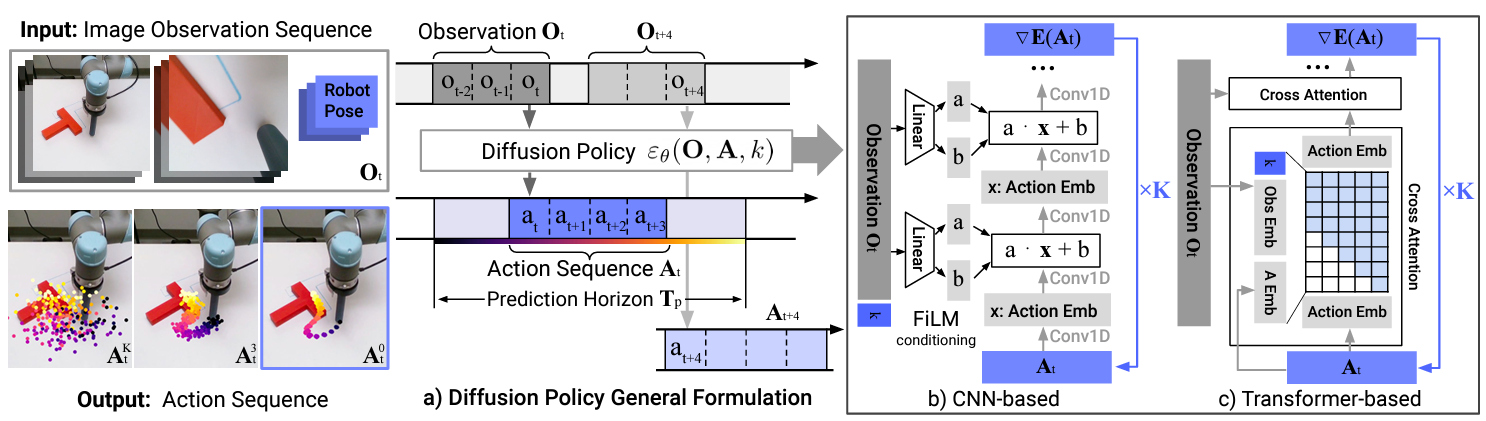### Mathematical background

We explain why the Diffusion Policy formulation is effective in dealing with discreteness and multimodality and in stabilizing learning.

First, the process of learning noise in a diffusion process can be considered equivalent to estimating scores in ( see here for details)Then, considering this score in the current problem setting, it can be expressed as the derivative of the log-likelihood of the probability of action generation that appeared in Implicit Policy, $\nabla_{\boldsymbol{a}} \mathrm{log} \hspace{2pt} p(\boldsymbol{a}|\boldsymbol{o})$ can be expressed as $(\boldsymbol{a}|{boldsymbol{o})$. In this case, the score can be expressed asand the term that had to be approximated by a sample can be calculated, eliminating the need for sample approximation and making the learning process more stable.

The figure below shows the correspondence between the Implicit Policy and the Diffusion Policy. The Diffusion Policy considers something like the gradient field of the Implicit Policy.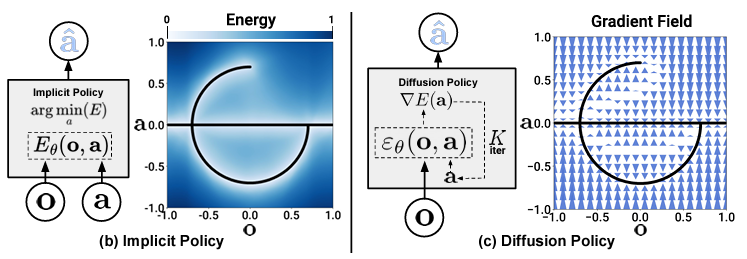### Special characteristic

We check that the desired characteristics are actually obtained, based on the mathematical background.

The figure below shows the trajectory taken by the robot arm to reach the target position and orientation of the T-junction block. In this case, since the robot can move toward the T-block from either the left or right side, there are roughly two possible solutions.The figure below shows the prediction error of the action and the success rate of the task during the learning process for the task of pushing the T-block. The superiority of not using sample approximation is demonstrated.## Experiment

Simulation and actual experiments are conducted using the Diffusion Policy. Here, actions are commands for robot hand positions and velocities, and observations are embedding robot joint information and image information. The video on this site will give you a better idea.

### Simulation Experiment

Comparisons are made with conventional methods in a variety of simulation environments and tasks. Examples of tasks and results are shown below. Although the results of the conventional method are based on the best performance of each learning process, Diffusion Policy significantly outperforms it in all tasks, improving its success rate by an average of 46.9%.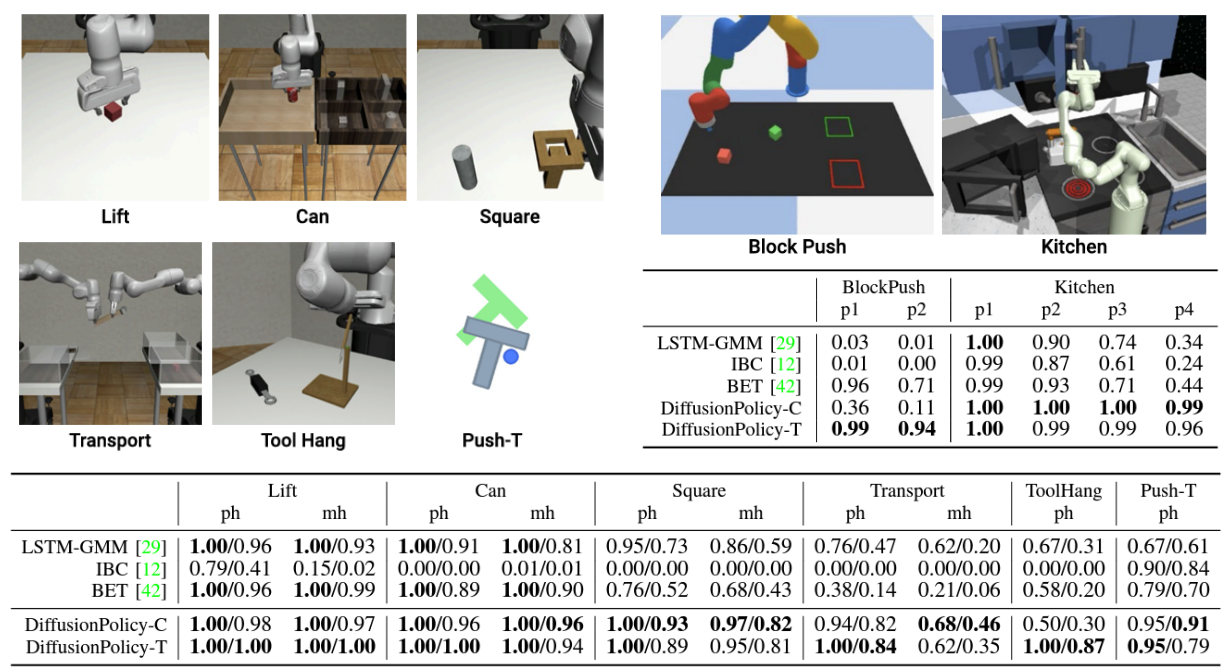### Experimental equipment

Two of the experiments we are conducting are introduced below.

In the task of moving the T-junction block to the target position and orientation, the success rate of 95% was achieved, far exceeding the success rate of conventional methods (see figure below). As the video shows, the T-block controller is extremely robust, as evidenced by the fact that it does not move even slightly even when a human blocks the camera and that it immediately corrects to the target position and orientation even after the block is moved.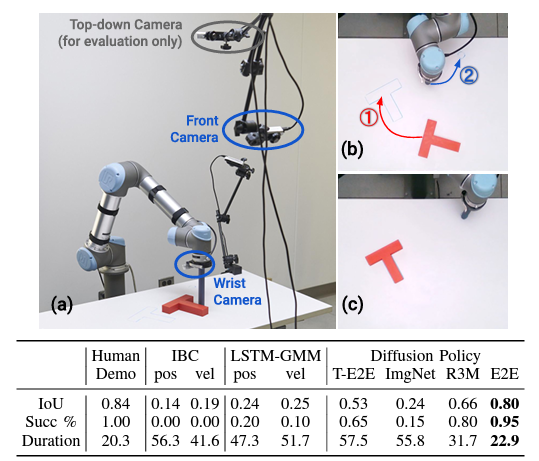The task of making a pizza is also challenging. Diffusion Policy has demonstrated human-like performance in non-rigid and fluid tasks such as this. The success rate of conventional methods is close to 0%, failing to separate a series of actions into discrete action sequences (left ①-④), whereas Diffusion Policy is able to separate action sequences and achieves a high success rate.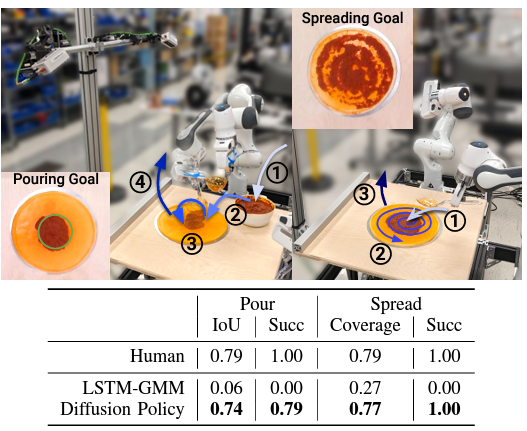## Summary

In this research, Diffusion Policy using the Diffusion Model was proposed to solve the problems of discreteness and multimodality of Explicit Policy and unstable learning of Implicit Policy. We have confirmed that the Diffusion Policy has a mathematical background and has actually solved the problems we have faced so far. We have conducted all kinds of experiments, from simulations to real-world environments, and obtained results that far exceed those of previous studies.

Personally, I believe that the high expressive power of the diffusion model is one of the reasons for this level of performance, in addition to the mathematical aspect of stabilizing the learning of Implicit Policy, of course. I feel that we are approaching a world like science fiction, which I thought was a dream, and I look forward to its further development in the future.

If you have any suggestions for improvement of the content of the article,